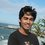# Minimum value of a function

I want to know how to calculate the minimum value of the following function and similar functions:

$y = \sqrt{(x^2+8x+25)}+ \sqrt{(x^2-8x+25)}$

I know I can just plot the graph in a calculator or computer, but is there any way to calculate it manually? Can somebody help me with this/suggest some resource?Note by Labib Rashid
6 years, 9 months ago

This discussion board is a place to discuss our Daily Challenges and the math and science related to those challenges. Explanations are more than just a solution — they should explain the steps and thinking strategies that you used to obtain the solution. Comments should further the discussion of math and science.

When posting on Brilliant:

• Use the emojis to react to an explanation, whether you're congratulating a job well done , or just really confused .
• Ask specific questions about the challenge or the steps in somebody's explanation. Well-posed questions can add a lot to the discussion, but posting "I don't understand!" doesn't help anyone.
• Try to contribute something new to the discussion, whether it is an extension, generalization or other idea related to the challenge.

MarkdownAppears as
*italics* or _italics_ italics
**bold** or __bold__ bold
- bulleted- list
• bulleted
• list
1. numbered2. list
1. numbered
2. list
Note: you must add a full line of space before and after lists for them to show up correctly
paragraph 1paragraph 2

paragraph 1

paragraph 2

[example link](https://brilliant.org)example link
> This is a quote
This is a quote
    # I indented these lines
# 4 spaces, and now they show
# up as a code block.

print "hello world"
# I indented these lines
# 4 spaces, and now they show
# up as a code block.

print "hello world"
MathAppears as
Remember to wrap math in $$ ... $$ or $ ... $ to ensure proper formatting.
2 \times 3 $2 \times 3$
2^{34} $2^{34}$
a_{i-1} $a_{i-1}$
\frac{2}{3} $\frac{2}{3}$
\sqrt{2} $\sqrt{2}$
\sum_{i=1}^3 $\sum_{i=1}^3$
\sin \theta $\sin \theta$
\boxed{123} $\boxed{123}$

Sort by:

Think this as the sum of distance from the point (x,0) to (4,3) and (-4,-3). To minimize, (x,0) has to be on the line passing through (4,3) and (-4,-3).

- 6 years, 9 months ago

Using $2$ Right angle $\triangle$, Like $\sqrt{a^2+b^2}+\sqrt{c^2+d^2}\geq \sqrt{(a+b)^2+(c+d)^2}$

Where $a,b$ denote base and height of one $\triangle$ and $c,d$ denote base and height of other $\triangle$

and these $2-\triangle$ are in opposite directions. and equality hold when $\displaystyle \frac{a}{b} = \frac{c}{d}$

So $\sqrt{(x+4)^2+3^2}+\sqrt{(4-x)^2+3^2}\geq \sqrt{(x+4+4-x)^2+(3+3)^2}= 10$

and equality hold when $\displaystyle \frac{x+4}{3} = \frac{4-x}{3}\Rightarrow x = 0$

- 6 years, 9 months ago

Thanks. Could you suggest where I can find some resources on the idea you used here?

- 6 years, 9 months ago

As far as I can tell, he used Minkowski's inequality of square root sums. But I think George G.'s method is better.

- 6 years, 8 months ago

Generally, differentiate and set equal to zero. For this one I got a minimum at x=0 and y=10. So I conjecture that any equation in this form (just swap out both the eights or both the 25's for other numbers) will have a minimum at x=0.

- 6 years, 9 months ago

We can write,

$y=\sqrt{(x+4)^2+(0+3)^2} + \sqrt {(x-4)^2+(0-3)^2}$

Let $A=(x,0) ; B=(-4,-3) ; C=(4,3)$

So, $y=AB+AC$

Using Triangular Inequality, $AB+BC \geq BC = \sqrt{(4+4)^2+(3+3)^2}=10$

So the minimum value of $y$ is $\fbox{10}$

- 5 years, 7 months ago

just squaring on both times twice we can get a quadratic equation ..this is an alternate way to solve this problem and but it is a tedious one.

- 6 years, 9 months ago

differentiate the function and equate it to zero. The value of 'x' is the local minima.

- 6 years, 9 months ago